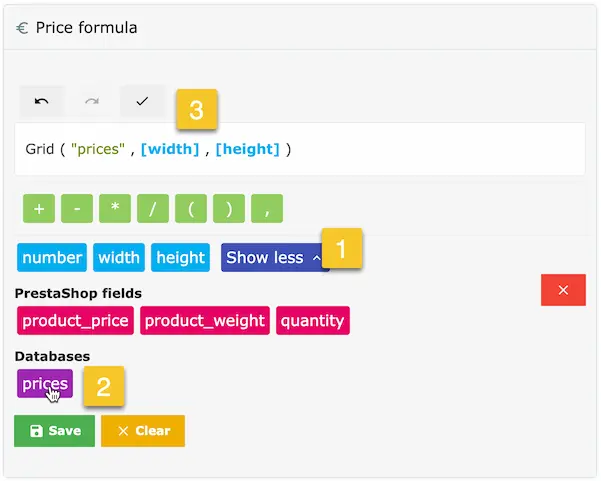# # Formula functions

The module supports many Excel functions that you can use in your calculations

## # Standard functions

### #SQR

Return the square of a number

``````SQR(5) → 25
``````

### #SQRT

Returns the square root of a number

``````SQRT(25) → 5
``````

### #ABS

Returns the absolute value of a number

``````ABS(-10) → 10
``````

### #SIGN

Returns the signs of a number

``````SIGN(10) → 1
SIGN(-10) → -1
SIGN(0) → 0
``````

### #TRUNC

Returns the truncated number

``````TRUNC(4.9) → 4
TRUNC(-3.5) → -3
``````

### #CEIL

Rounds a number up

``````CEIL(3.2) → 4
CEIL(-3.14) → -3
``````

### #FLOOR

Rounds a number down

``````FLOOR(3.2) → 3
FLOOR(-3.14) → -4
``````

### #ROUND

Uses PrestaShop rounding method (configurable in the PrestaShop settings)

``````ROUND(number, decimals)
ROUND(5.16, 0) → 5
ROUND(5.16, 1) → 5.2
``````

### #ROUNDUP

Rounds a number up using PrestaShop's rounding method

``````ROUNDUP(number, decimals)
ROUNDUP(5.16, 0) → 6
ROUNDUP(5.11, 1) → 5.2
``````

### #ROUNDDOWN

Rounds a number down using PrestaShop's rounding method

``````ROUNDDOWN(number, decimals)
ROUNDDOWN(5.16, 0) → 5
ROUNDDOWN(5.11, 1) → 5.1
``````

### #VAL

Returns the number value of a string

``````VAL("2") → 2
``````

### #POW

Raise a number to a specific power

``````POW(5, 3) → 125
``````

### #MIN

Return the smallest value among the passed parameters (accept two parameters or more)

``````MIN(10, 5, 14, 3) → 3
``````

### #MAX

Return the biggest value among the passed parameters (accept two parameters or more)

``````MAX(10, 5, 14, 3) → 14
``````

### #IF

Test for a specific condition

``````IF(condition, value if true, value if false)
IF(100 > 10, 1, 2) → 1
IF(5 > 10, 1, 2) → 2
``````

### #STRLEN

Return the number of characters in a string (supports UTF-8)

``````STRLEN('Hello') → 6
STRLEN('World') → 5
``````

### #CHECK

Checks if a string if not empty, return 0 if string is empty, 1 otherwise

``````CHECK("Hello") → 1
CHECK("") → 0
``````

### #SUBSTR

Extract a string from another string

``````SUBSTR(string, start, length)
SUBSTR("Hello world!", 0, 6) → "Hello"
``````

### #REPLACE

Find and replace a string in another string

``````REPLACE(string, find, replace)
REPLACE("It's a good day", "good", "great") → "It's a great day"
``````

### #CONCAT

Joins multiple strings together, accepts two strings or more

``````CONCAT("A great ", "day") → "A great day"
CONCAT( "A great ", "day ", "today" ) → "A great day today"
``````

## # The Grid function

Grid: Allows reading a CSV file and getting a result based on two values
This function is very similar to the Grid feature.

Here's a CSV sample

To use this function, start by uploading your CSV file to the folder `[root of PrestaShop]/dynamicproduct/databases/`
Then in the formula, you can insert this function by clicking the CSV file

1. Click the "Show more" button
2. Click the CSV file to insert the Grid function into the formula
3. Insert the two other fields that will act as the input to the grid

In this case, we get a price based on the width and height valuesThis method allows you to use a single CSV file for multiple products

TIP

For decimal numbers, use the dot instead of the comma.
For example, write `10.5` instead of `10,5`

Last Updated: 4/21/2021, 12:37:35 PM